• 哈夫曼算法证明 哈夫曼算法是一种贪心算法，我们考虑证明其最优子结构和贪心选择性质： 最优子结构：假设一个树是哈夫曼树，则以其任意节点为根节点的最大子树也是哈夫曼树。 证明：子树的根节点的值是其所有叶子...
哈夫曼算法证明
哈夫曼算法是一种贪心算法，我们考虑证明其最优子结构和贪心选择性质：

最优子结构：假设一个树是哈夫曼树，则以其任意节点为根节点的最大子树也是哈夫曼树。
证明：子树的根节点的值是其所有叶子节点出现权值之和，因此无论子树是什么形式，对子树上方的节点计算WPL2都没有影响。
根据哈夫曼树的定义：WPL最小的二叉树。如果子树不是哈夫曼树，其WPL1就不会是最小，那么整个树的WPL=WPL1+WPL2就不会是最小，这与哈夫曼树的定义相悖，因此子树是哈夫曼树。

贪心选择性质（哈夫曼算法）：每次去掉权值最低的两个节点作为叶子节点形成一颗二叉树，并将其父亲节点放入待选节点中。
证明：对于哈夫曼树我们总可以通过取出两个节点作为叶子节点并将其父亲节点重新作为叶子节点的方式构造，需要证明的是是否每次选择权值最低的节点可以构造成功。
当含有2个及以下的叶子节点的时候，显然正确。
假设当含有小于k个叶子节点的时候哈夫曼算法可以形成哈夫曼树
对于含有k个叶子节点形成的树，设其中权重最小的叶子节点为a,其中深度最深的叶子节点为b，h表示节点的深度，w表示节点的权值。则：
$WPL_1=\sum+w_a*h_a+w_b*h_b$
交换这两个节点：
$WPL_2=\sum+w_b*h_a+w_a*h_b$

$WPL_1-WPL_2=(w_a-w_b)*h_a+(w_b-w_a)*h_b=(h_b-h_a)*(w_b-w_a)$
而$h_b-h_a$和$w_b-w_a$都为正（由a,b节点的性质），所以我们得到结论：对于任何一棵树，将权值小的节点尽可能移动到较深层的节点会使整个树的WPL比较小。
对于k个节点，我们首先取出两个节点，将其合并成一个节点以后我们有k-1个节点，可以使用哈夫曼算法构造。
如果这两个节点不是所有节点中权值最小的两个，则我们总可以通过交换使得构造的树的WPL减小，因此不是哈夫曼树。只有两个节点是所有节点中权值最小的两个时我们无法再降低树的WPL。因为我们总可以构造成功，所以选择权值最小的节点构造的树就是哈夫曼树。证毕。
哈夫曼编码译码程序
#pragma once
#include<iostream>
#include<fstream>
#include<vector>
#include<queue>
#include<cstdio>
#include<string>

using namespace std;

static const int MAXN = 1005;

struct times
{
double weight;//字符的权值
int num;//字符的序号，同时也是他的ASCALL值
times(int _num=0,double _weight=0) :num(_num),weight(_weight){}
friend bool operator < (const times & a,const times & b)
{
return a.weight > b.weight;
}
}p,q;

struct Chara:times
{
int father;//父亲的序号
int lson, rson;//左右儿子的序号
string code;
Chara(int _num = 0, double _weight = 0, int _lson = 0, int _rson = 0, int _father = 0) :times(_num,_weight), lson(_lson), rson(_rson), father(_father)
{
code = "";//没有编码
}
void operator = (const Chara& x)
{
weight = x.weight; num = x.num; father = x.father; lson = x.lson; rson = x.rson;
}
};

struct txt
{
string t;
double weight;
txt(string _t,double _weight):t(_t),weight(_weight){}
};

class HuffmanCode
{
public:
int n=0;//字符的个数
int cur;//当前所在位置
int root;//哈夫曼树根节点
Chara A[MAXN];//顺序表保存哈夫曼树
priority_queue<times> T;//用来构建哈夫曼树
void CreatHuffmanCode(int x, string now)
{
if (A[x].lson != 0)
{
CreatHuffmanCode(A[x].lson, now + "0");
}
if (A[x].rson != 0)
{
CreatHuffmanCode(A[x].rson, now + "1");
}
if (A[x].lson == 0 && A[x].rson == 0)//说明是字符
{
A[x].code = now;
}
}

void _HuffmanCode(int _n,vector<txt>& input)
{
string tmp;
n = _n;
for (int i = 0; i < n; i++)
{
tmp = input[i].t;
A[tmp].num = tmp;
A[tmp].weight = input[i].weight;
T.push(times(tmp, A[tmp].weight));
}
cur = 500;

//构建哈夫曼树
while (T.size() > 1)
{
p = T.top(); T.pop(); q = T.top(); T.pop();
A[cur] = Chara(cur, p.weight + q.weight, p.num, q.num, 0);
A[p.num].father = A[q.num].father = cur;
T.push(times(A[cur])); cur++;
}
T.pop(); root = cur-1;

CreatHuffmanCode(root, "");
}
};

class Huffman
{
int n;
vector<txt> input;
HuffmanCode x;
public:
void _Huffman()
{
string t; double weight; n = 0;
FILE* stream;
freopen_s(&stream,"hfmTree.txt","r",stdin);
while (1)
{
cin >> t;
if (t == "Esc") break;
n++;
cin >> weight;
input.push_back(txt(t, weight));
}
freopen_s(&stream, "CON", "r", stdin);
input.push_back(txt(" ", 10000.0));//给空格很大的权值
n++;
x._HuffmanCode(n, input);
}
Huffman()
{
string t; double weight; n = 0;
cout << "请输入字符集 \n[Delete撤销输入,Esc退出输入]\n[直接输入Default按照默认文件组成哈夫曼编码]\n[空格已经编码]"<< endl;

while(1)
{
cout << "请输入字符：";
cin >> t;
if (n == 0 && t == "Default")//按照默认文件构造哈夫曼树
{
_Huffman();
return;
}
else if (t == "Delete")
{
input.erase(input.end()-1);//删除最后一个输入的字符
n--;
continue;
}
else if (t == "Esc")
{
break;
}
n++;
cout << "请输入" << t << "的权重：";
cin >> weight;
input.push_back(txt(t, weight));
}
FILE* stream;
freopen_s(&stream, "hfmTree.txt", "w", stdout);
for (int i = 0; i < n; i++)
{
cout << input[i].t << " " << input[i].weight << endl;
}
cout << "Esc" << endl;
freopen_s(&stream, "CON", "w", stdout);
input.push_back(txt(" ", 10000.0));//给空格很大的权值
n++;
x._HuffmanCode(n, input);
}
void HuffmanDisplay()
{

cout << "哈夫曼编码：" << endl;
for (int i = 0; i < n; i++)
{
cout << input[i].t << ":" << x.A[input[i].t].code << endl;
}
}
void GenerateCode()//压缩文件
{
cout << "请输入需要压缩文件的路径[输入Default将打开默认文件ToBeTran.txt]" << endl;
string in;

cin >> in;
if (in == "Default")
{
in = "ToBeTran.txt";
}
cout << "请输入保存压缩后文件的路径[输入Default将打开默认文件CodeFile.txt]" << endl;
string out;

cin >> out;
if (out == "Default")
{
out = "CodeFile.txt";
}

ifstream infile(in, ios::in);
ofstream outfile(out, ios::out);

char c;
bool flag = true;
while ((c = infile.get()) != EOF)
{
if (x.A[c].code == "")
{
flag = false;
break;
}
outfile << x.A[c].code;
}

infile.close();
outfile.close();

if (!flag)
{
cout << "压缩失败，文件中出现了字符集中未包含的字符" << endl;
return;
}

//展示压缩的结果:
infile.open(out, ios::in);

string tmp;
infile >> tmp;

infile.close();

cout << "编码后的文件为：" << endl;

for (int i = 0; i < tmp.size(); i++)
{
if (i && i % 50 == 0) cout << endl;
cout << tmp[i];
}
cout << endl;

//将结果放入文件中
outfile.open("CodePrint.txt", ios::out);

for (int i = 0; i < tmp.size(); i++)
{
if (i % 50 == 0) outfile << endl;
outfile << tmp[i];
}

outfile.close();
}
void Decode()
{
cout << "请输入需要解码文件的路径[输入Default将打开默认文件CodeFile.txt]" << endl;
string in;
cin >> in;
if (in == "Default")
{
in = "CodeFile.txt";
}
cout << "请输入保存压缩后文件的路径[输入Default将打开默认文件TextFile.txt]" << endl;
string out;

cin >> out;
if (out == "Default")
{
out = "TextFile.txt";
}

ifstream infile(in, ios::in);

string ss;
infile >> ss;
//cout << "test:" << ss << endl;
int i = 0;
string sss;

while (i < ss.size())
{
int t = x.root;
while ((x.A[t].lson != 0 || x.A[t].rson != 0) && i < ss.size())
{
if (ss[i] == '0') t = x.A[t].lson;
else t = x.A[t].rson;
i++;
}
if (x.A[t].lson == 0 || x.A[t].rson == 0)
{
sss = sss + (char)t;
}
}

infile.close();

cout << "解码后为：" << endl;
cout << sss << endl;

ofstream outfile(out,ios::out);

outfile << sss << endl;

outfile.close();
}
};

测试代码
#include"Huffman.h"

#include<iostream>

using namespace std;

int main()
{

Huffman x;

x.HuffmanDisplay();

x.GenerateCode();

x.Decode();

}


测试结果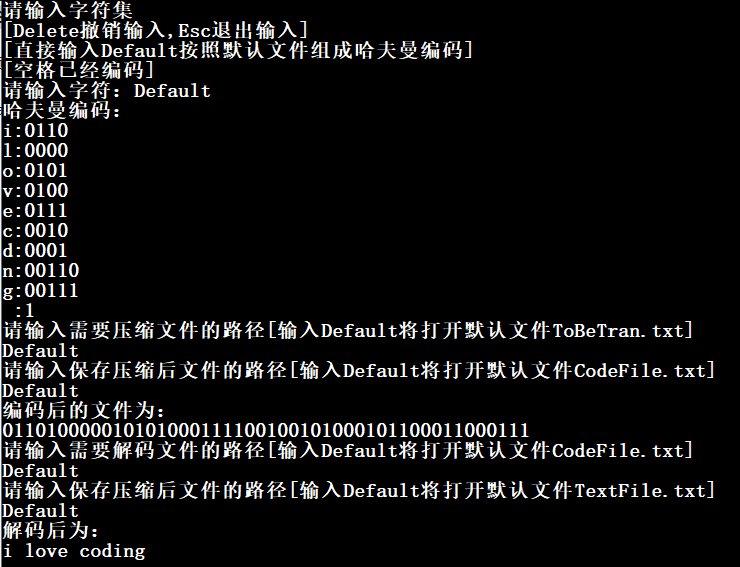展开全文• 哈夫曼算法最优哈夫曼树是啥算法步骤简介复杂度算法正确性证明 最优哈夫曼树是啥 有篇文章（字符串），想把它加密成01串。所以要给每个字符映射一个01串代表它，而且一个字符的01串不能是另一个的前缀，否则将出现二...
哈夫曼算法最优哈夫曼树是啥算法步骤简介复杂度算法正确性证明
最优哈夫曼树是啥
有篇文章（字符串），想把它加密成01串。所以要给每个字符映射一个01串代表它，而且一个字符的01串不能是另一个的前缀，否则将出现二义性。所以可以把一颗二叉树的叶子节点看成字符，向左走和向右走分别为0和1，这样构造映射到的01串就不会有二义性，这个树就是哈夫曼树。为了使得01串总长度最小，就要构造最优哈夫曼树。显然每个字符的01串长度是字符节点的深度（到根节点经过的变数），所以使得$len=\sum{cnt_i*deep_i},i\in\sigma$
最小。
其中$cnt_i,deep_i,\sigma$分别表示字符i出现的次数，字符i的叶子结点深度，字符集合。以下n是字符集大小。
算法步骤简介
采用贪心算法。一开始节点集合有n个字符节点，每个节点权为字符权重。每次选择剩下的节点中选两个权重最小的，合并成一个新节点，权重为两节点之和。删除两个节点，移入新节点。旧的两个节点就是新节点的左右儿子。知道集合里只有一个节点。
复杂度
每次集合减少一个点，用优先队列模拟选点过程的话，时间为$T=\sum_{i=2}^{n}log_2i+log_2(i-1)=O(nlogn)$
算法正确性证明
众所周知，贪心算法要满足最优子结构和第一步正确性。

所以我就从这两方面下手:
1.第一步正确性证明：
令n个结点中最小的两个是x,y。就是证明们对于n个叶子节点的哈夫曼树，开始选x,y合并是可以构造可以出哈夫曼树的。即证明n个叶子节点的最优哈夫曼树集合中，一定有一棵，x,y处于层数最深的那层，且互为兄弟。

(1) 层数最深证明：
现在有一棵按算法构造的树T，加密01串总长				     度len。如果把层数比较浅的叶子z节点（按算法$cnt_z>=cnt_x$）和  				x交换，那么新的总长度$len'=len+(deep_z-deep_x)*cnt_z-(deep_z-deep_x)*cnt_x\\ =len+(deep_z-deep_x)*(cnt_z-cnt_x)\\ \ge len$
所以交换深度一定不会让总长度变小。所以x,y一定层数最深的这棵树一定是最优的。

(2) x,y是兄弟证明：同层交换不改变总长度。所以某棵树xy不在一起的话，根据层数最深规则xy一定在同一层。所以设y现在的兄弟是z，把x和z换一下最优性不变而使得xy互为兄弟。所以一定有一棵最优树x,y互为兄弟。

到这里第一步正确性就成立了。

最优子结构：
这个比较好办。现在是n个点，弄完第一步后剩n-1个点，要证明这n-1个点也得构成最优树才能保证n个点也是最优树。证明：
设n-1个点构成了T‘树，总长度为len’。此时n个点的树的$len=len‘+cnt_x+cnt_y$显然len’最优才能保证len最优。

所以满足最优子结构和第一步正确性，算法是合理的。


展开全文• 复杂度分析：初始化极小堆时需计算时间O(n)，其函数remove() 和put() 运算时间需O(logn)，总共n-1次合并需要时间O(nlogn)，故哈夫曼算法的时间复杂度为  T(n)=O(nlogn)   3、贪心选择性质  已知 c 是字符...
编码方式：
1）定长码：每个不同字符的编码长度是规定的，译码是唯一的。
2）变长码：每个不同字符的编码长度不同，比定长码方案好，但译码困难。

前缀码：
1）定义：对任一字符的一个0,1串编码，都不是其他字符编码的前缀
2）作用：前缀性质可以使译码方法非常简单
3）表示：用完全二叉树来表示，即树中任一结点都有2个儿子结点

平均码长：其中C表示字符集，c表示任意字符，f(c)表示字符出现的频率，也即权值，dT(c)表示前缀码长。

最优前缀码：使平均码长达到最小的前缀码编码方案称为给定编码字符集C。

1、构造哈夫曼树

1）根据给定的n个权值 ，构造n棵只有一个根结点的二叉树， n个权值分别是这些二叉树根结点的权。设F是由这n棵二叉树构成的集合；
2）在F中选取两棵根结点树值最小的树作为左、右子树，构造一颗新的二叉树，置新二叉树根的权值=左、右子树根结点权值之和；
3）从F中删除这两颗树，并将新树加入F；
4）重复步骤 2、3，直到F中只含一颗树为止。

贪心策略：每次选择权值最小的子树构造新的二叉树。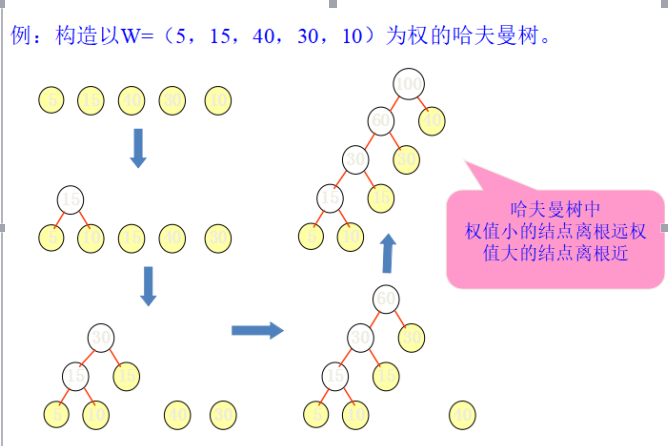2、哈夫曼算法

/*定义树的结构体*/
private static class Huffman implements Comparable{
Bintree tree;
static float weight;
private Huffman(Bintree tt,float ww){
tree=tt;
weight=ww;  //权重
}
public int comoareTo(Object x){
float xw=((Huffman)x).weight;
if(weight<xw)
return -1;
if(weight==xw)
return 0;
}
}
/*构造哈夫曼树的算法，其中f[]为字符权值数组*/
private static Bintree huffmanTree(float[]f){
//生成只有根结点的单结点树
int n=f.length;
Huffman[]w=new Huffman [n+1]; //定义二叉树的结构
Bintree zero=new Bintree();   //构造空子树
for(int i=0;i<n;i++){
Bintree x=new Bintree();
x.makeTree(new MyInteger(i),zero,zero); //左右孩子均为空
w[n+1]=new Huffman(x,f[i]); // 刻画根结点的权值
}
MinHeap H=new MinHeap();  // 创建极小堆
H.initialize(w,n);    //堆初始化
/*反复合并最小权值的子树*/
for(int i=0;i<n;i++){
Huffman x-(Huffman)H.removeMin();   //取出堆顶元素，即最小元素x，并调整堆
Huffman y-(Huffman)H.removeMin();   //继续取出堆顶元素，即次小元素y，并调整堆
Bintree z=new Bintree();  //定义根结点
z.makeTree(null,x.tree,y.tree);  //构造具有左右孩子 x,y 的子树 z
Huffman t=new huffman(z,x,weight+y,weight); //计算父结点z的权值
H.put(t);  //将根的权值插入堆
}
return ((Huffman)H.removeMin()).tree; //返回生成树，即哈夫曼树
}

复杂度分析：初始化极小堆时需计算时间O(n)，其函数remove() 和put() 运算时间需O(logn)，总共n-1次合并需要时间O(nlogn)，故哈夫曼算法的时间复杂度为 T(n)=O(nlogn)

3、贪心选择性质

已知 c 是字符集，f(x)≤f(c) , f(y)≤f(c)，且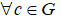证明：存在 T''，使得（ x 与 y 的码长相等），且 x 与 y 为兄弟（权值最小），当 x,y 在任何位置时，需要证明 B(T'') ≤ B(T') ≤ B(T)。如下图：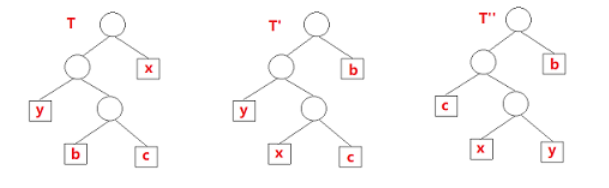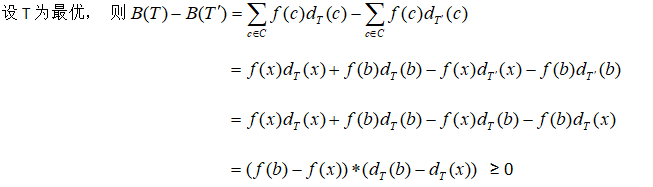所以 B(T') ≤ B(T)，同理可证B(T'') ≤ B(T') ==> B(T'') ≤ B(T') ≤ B(T) ==> B(T'') ≤ B(T)，由假设B(T) ≤ B(T'') ==> B(T) = B(T'')

4、最优子结构性质

已知T'为最优，x,y 为叶结点且同为兄弟，z为其父亲，则f(z) = f(x) + f(y)，T = T'- {x,y} U {z}证明：T'= T- {x,y} 为c’ = c - {x,y} U {z}，所以T' 表示字符集c’ 的一个最优前缀码，需证明 B(T)=B(T')+f(x)+f(y) 且T' 是c’ 的最优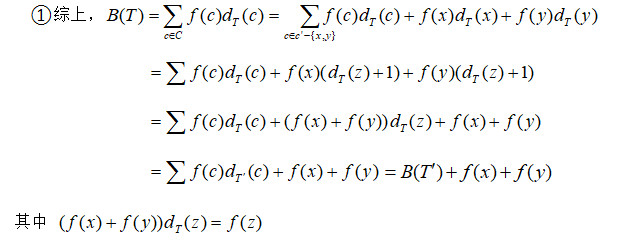②反证：假设T'不是最优，而T''是最优，则

B(T''') = B(T'')+f(x)+f(y) ≤ B(T')+f(x)+f(y) = B(T)，即B(T''') ≤ B(T)，得证T'''为最优，与假设T'' 最优矛盾。如下图：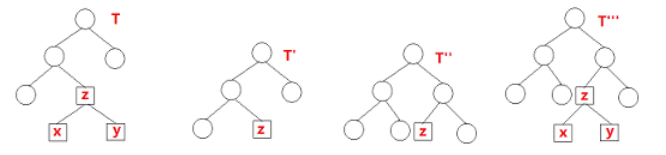展开全文• 21个项和10个项的文件归并，比较一次拿走一个，最后剩下一个可以不用比较。 21这个文件里的每一个项，在它上边要参与3次归并，每次归并，这里面的项都要参与一次比较。也就是说，21这个结点里面的每一个项都要...
• 提出了一种改进的四进制哈夫曼树的生成算法，通过分析算法的平均码长和编码效率，论证了算法相对于传统的四进制算法的优点。并用C语言分别实现两种算法，进行了压缩比和压缩时间的比较，证明了改进算法在压缩比和...数据压缩
• 数据结构与算法—复习：最优二叉树 哈夫曼算法 手画很简单，代码则需要多考虑 /** * 程序说明：复习 最优二叉树（哈夫曼树） 哈夫曼算法 */ #include <stdio.h> #include <malloc.h> /** * 定义一...数据结构
• 摘要 当一个问题具有最优子结构性质时，可用动态规划...关键词 贪心算法,贪心选择性质,最优子结构性质,哈夫曼算法,三元码 1问题简述哈夫曼编码是广泛地用于数据文件压缩的十分有效的编码方法。其压缩率通常在20%～...贪心算法
• 哈夫曼树 在一般的数据结构的书中，树的那章后面，著者一般都会介绍一下哈夫曼(HUFFMAN)树和哈夫曼编码。哈夫曼编码是哈夫曼树的一个应用。哈夫曼编码应用广泛，如JPEG中就应用了哈夫曼编码。 首先介绍什么是...哈夫曼 哈夫曼树 最优二叉树
• 根据每个字符的出现频率，哈夫曼贪心算法构造出字符最优的二进制表示。 假定我们希望压缩一个十万个字符的数据文件，设文中只有6个不同字符，每个字符的频次、定长编码、变长编码如下表所示： 信息 a b c d e...数据结构
• 问题 ...现在我们要对a,b,c,d,e,f,g 7个字符进行编码，一种笨办法...总结下来就是，靠__不定长编码__节省了空间，并且靠__不同前缀__保证了非歧义性，同时Huffman又证明了这样生成的确实是最优二叉树
• 首先介绍什么是哈夫曼树。哈夫曼树又称最优二叉树，是一种带权路径长度最短的二叉树。所谓树的带权路径长度，就是树中所有的叶结点的权值乘上其到根结点的路径长度（若根结点为0层，叶结点到根结点的路径长度为叶...网络应用 F# C C++
• 哈夫曼编码与哈夫曼算法 哈弗曼编码的目的是，如何用更短的bit来编码数据。 通过变长编码压缩编码长度。我们知道普通的编码都是定长的，比如常用的ASCII编码,每个字符都是8个bit。但在很多情况下，数据文件中的...
• 下面我们用数学归纳法证明 数学归纳法证明哈夫曼算法证明一个性质1：C是字符集，x,y∈C，f(x),f(y)频率最小，那么存在最优二元前缀码使得x,y码字等长且仅在最后一位不同 这个翻译过来就是x,y是最深叶子结点且还是...java
• 本文内容为北大慕课课程的算法分析与设计的课程讲义,将其整理为OneNote笔记同时添加了本人上课时的课堂笔记,且主页中的思维导图就是根据课件内容整理而来, 为了方便大家和自己查看，特将此上传到CSDN博文中, 源文件...
• 然而，还有一种就是大家在学习数据结构最常见到的哈夫曼树的数据结构，以前还不知道他又什么用，其实他最大的用途就是用来做压缩，也是一些rar,zip压缩的祖先，称为哈弗曼压缩（什么你不知道谁是哈弗曼，也不知道...string byte 存储 search
• 哈夫曼树构造1．哈夫曼树的定义给定n个权值作为n个叶子结点，构造一棵二叉树，若带权路径长度达到最小，称这样的二叉树为最优二叉树，也称为哈夫曼树(Huffman tree)。 2．哈夫曼树的构造假设有n个权值，则构造出的...
• ## 算法学习之哈夫曼编码算法

万次阅读 多人点赞 2017-04-04 12:16:03
哈夫曼编码算法用字符在文件中出现的频率表来建立一个用0，1串表示各字符的最优表示方式。一个包含100,000个字符的文件，各字符出现频率不同，如下表所示。  有多种方式表示文件中的信息，若用0,1码表示...
• 以前的作业，拿出来看看，都不会了。郁闷记得当时为了完成这作业，求了一圈朋友，...课程目的是为了了解哈夫曼算法的思路核心，掌握哈夫曼算法在压缩算法中的基本应用，全面提高程序设计开发能力，并将程序应用于现...
• 博主自己手撸的代码，若有有错误，感谢指出直接上代码 目录 0 讲义 0.1 二元前缀码 0.2 平均传输位数 0.3 伪码 0.4 实例 1 代码 ...代码：哈夫曼编码 ...代码：生成哈夫曼树&...哈夫曼编码是数据...python 数据结构
• 哈夫曼树 哈夫曼树又称最优二叉树，是一种带权路径长度最短的二叉树。所谓树的带权路径长度，就是树中所有的叶结点的权值乘上其到根结点...哈夫曼算法原理： 1.为每个符号建立一个叶子节点，并加上其相应的发生频率
• 哈夫曼编码是通过构造哈夫曼树得到的， 哈夫曼树是通过贪心算法得到的； 贪心算法构造哈夫曼树： 将字符出现的次数作为哈夫曼树的权值，按照贪心算法，选择权值最小的两个结点，成为一个数的左右结点；左右子结点的...
• 淮海工学院计算机工程学院 实验报告书 课程名 算法分析与设计 题 目 实验3 贪心算法 哈夫曼...2掌握最优子结构性质的证明方法 3掌握贪心法的设计思想并能熟练运用 4证明哈夫曼树满足最优子结构性质 5设计贪心算法求解哈
• 简述：在学习慕课《数据结构》时，关于哈夫曼树仅给出了算法描述，并没有给出哈夫曼树就是最优树的证明，查阅教材也没发现相关信息，通过上网浏览博客解决了该问题。 参考博客：1）...
• 已知一组字符的频率，求其哈夫曼编码 即构造一棵哈夫曼树（字符均在叶子节点上） 如果使用固定编码会导致空间浪费，所以用哈夫曼编码减少浪费 分析 平均传输位数B=∑（字符出现的频率fX字符所在的叶子在书中的深度d...
• 哈夫曼编码算法用字符在文件中出现的频率表来建立一个用0，1串表示各字符的最优表示方式。一个包含100,000个字符的文件，各字符出现频率不同，如下表所示。  有多种方式表示文件中的信息，若用0,1码表示字符的...最小堆...